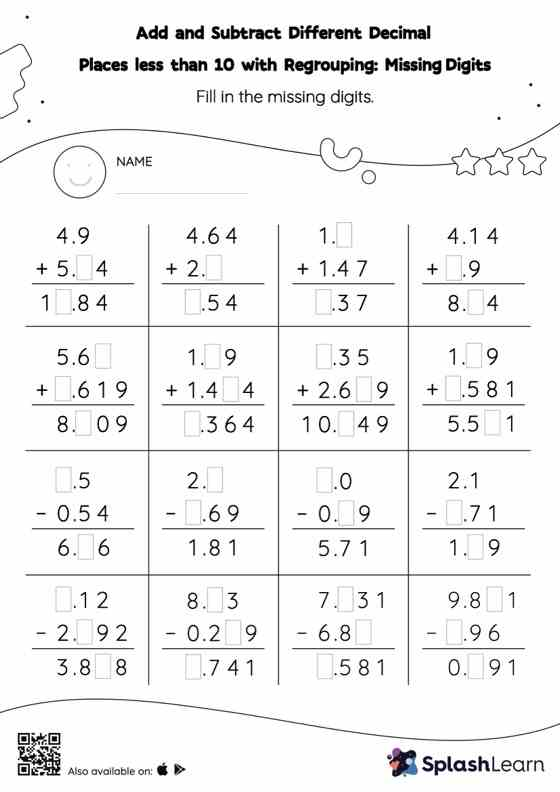# Add and Subtract Different Decimal Places less than 10 with Regrouping: Missing Digits Worksheet

Home > Add and Subtract Different Decimal Places less than 10 with Regrouping: Missing DigitsThis add and subtract different decimal places less than 10 with regrouping worksheet has a set of problems curated to ensure that your child becomes more proficient in addition and subtraction. In each problem, the numbers are laid out in the vertical format. In this format, pair of digits in each successive place are tackled one by one. This helps students follow a structured approach.